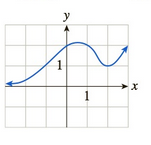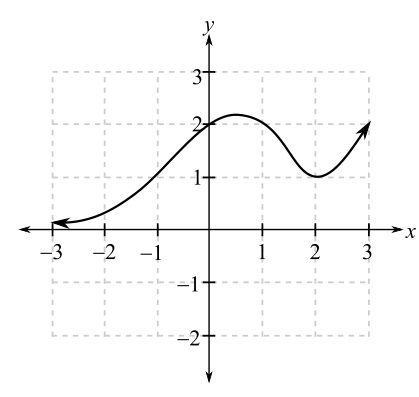Chapter 10.1, Problem 37EFinite Mathematics and Applied Cal...

7th Edition
Stefan Waner + 1 other
ISBN: 9781337274203

Solutions

Chapter
SectionFinite Mathematics and Applied Cal...

7th Edition
Stefan Waner + 1 other
ISBN: 9781337274203
Textbook Problem

In Exercises 35–48 the graph of f is given. Use the graph to compute the quantities asked for. [HINT: See Examples 4–5.]a. lim x → 0 f ( x ) b. lim x → 2 f ( x ) c. lim x → − ∞ f ( x ) d. lim x → + ∞ f ( x )(a)

To determine

The value of limx0f(x) using the graph which is as follows:Explanation

Given information:

The graph is as follows:

From the graph, limit is determined for x tends to 0 for the function f(x).

For this, take a point on the graph to the left of x=0 and move right along the curve, so that the x-coordinate approaches 0. Then, it is noticed from the graph that the y-coordinate approaches 2.

Similarly, take a point on the graph to the right of x=0 and move along the curve to the left so that the x-coordinate approaches 0

(b)

To determine

The value of limx2f(x) using the graph which is as follows:(c)

To determine

The value of limxf(x) using the graph which is as follows:(d)

To determine

The value of limx+f(x) using the graph which is as follows:Still sussing out bartleby?

Check out a sample textbook solution.

See a sample solution

The Solution to Your Study Problems

Bartleby provides explanations to thousands of textbook problems written by our experts, many with advanced degrees!

Get Started

Other Equations Find all real solutions of the equation. 98. 5x+1=x2

Precalculus: Mathematics for Calculus (Standalone Book)

In Exercises 33-38, rewrite the expression using positive exponents only. 36. x19x3

Applied Calculus for the Managerial, Life, and Social Sciences: A Brief Approach

True or False: i + j is a unit vector in the direction of 5i + 5j.

Study Guide for Stewart's Multivariable Calculus, 8th

For f(x) = tanh1 2x, f(x) = a) 2(sech1 2x)2 b) 2(sech2 2x)1 c) 214x2 d) 114x2

Study Guide for Stewart's Single Variable Calculus: Early Transcendentals, 8th